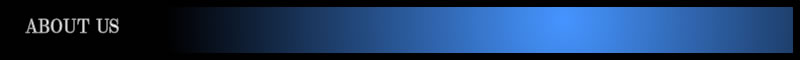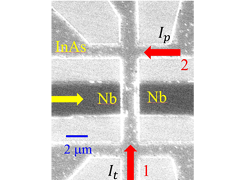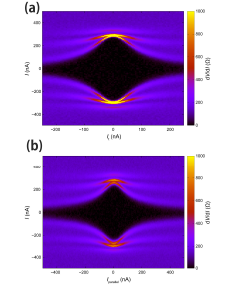# ISSP - The institute for Solid State PhysicsFont Size: (S) / (M) / (L)

- Activity Report 2018 -

Katsumoto Group

Realization of a Current-Controlled Superconducting DeviceFig. 1. Scanning electron micrograph of the sample. The yellow arrow indicates the supercurrent while two red arrows indicated the transverse (no.1) and the parallel (no.2) normal currents, respectively.Fig. 2. (a) Color plot of the junction resistance versus the plane of transverse current and junction current. The black region corresponds to the zero-resistance state. (b) The same for the parallel current.

Ultra-high speed, low-power consumption logic device action has been predicted for Josephson superconducting junctions, which dream, however, has not come true yet for more than three decades. The device action itself had been confirmed at the beginning of the 1980s . Nevertheless, the two-terminal nature of the junction has blocked the application to the real world of logic devices. The obstacle is explained as follows. The switching to normal state occurs when the junction is “fired” by applying a bias current larger than the critical current Jc (Jc1). Then to switch the next junction a current larger than its Jc (Jc2) is required while the current swing of the first junction is Jc1. Hence for the circuit action, condition Jc1 > Jc2 should be fulfilled. The condition means that Jc decreases monotonically over the chain of logic gates, thus sharply limits the scalability of the circuit. The finding of a gate-controllable supercurrent through the two-dimensional electrons (2DE) on the surfaces of InAs , this was thought to solve the problem. However, as a voltage-driven device, the Josephson junctions have too high off-conductance, and the obstacle was realized to exist stubbornly. Therefore a current-current three(or four)-terminal device is required for the practical use.

We here report pure current-control of supercurrent, which would solve the long-standing technical problem. We prepared a 2DE in an InAs quantum well grown on an InP substrate. Figure 1 shows the sample structure, in the center of which a Nb-InAs 2DE-Nb superconducting junction is formed. Besides, the sample has six normal terminals, through which local or non-local electric currents can flow. InAs 2DE is known to have a strong Rashba-type spin-orbit interaction (RSOI), and the layered structure is design to maximize the RSOI.

One of the expected device actions is described as follows. When a supercurrent is flowing between the two Nb electrodes, a normal current is applied transverse to the supercurrent. The normal current is associated with the spin Hall effect, which causes spin accumulation at the super-normal interfaces and breaks the time-reversal symmetry locally. The normal current can thus reduce the critical current of the superconducting junction. The other is a kind of "remote reduction," in which the normal current is applied parallel to the supercurrent but at a different part of the sample (red arrow no.2). In this case, the normal current causes the spin current, which should have a significant disorder in electron spins through the Zitterbewegung  and crosses the supercurrent. The disordered spin current again should lead to the reduction of the critical current.

In the experiments, the care was taken to avoid heating by the normal currents and the application of voltages due to the imbalance of the potentials at the normal electrodes. Figure 2(a) is a color plot of the resistance of the superconducting junction against the junction current and the control current. The black region corresponds to the zero-resistance state, and an apparent reduction of the critical current is observed. The current gain (the derivative of the edge line on the zero-resistance region) is larger than unity (0 dB) below 100 nA of the transverse current. The result of the remote-reduction experiment is shown in Fig. 2(b). The remote current also reduces the critical current through the sensitivity is lower, and the region for positive current gain (above 0 dB) is limited to just around the zero parallel currents.

In conclusion, we have realized a current-controlled four terminal superconducting device with finite current gains.

References

•  T. R. Gheewala, IEEE Trans. Electron Devices 27, 1857 (1980).
•  H. Takayanagi and T. Kawakami, Phys. Rev. Lett. 54, 2449 (1985).
•  Y. Iwasaki, Y. Hashimoto, T. Nakamura, and S. Katsumoto, Sci. Rep. 7, 7909 (2017).

Authers

• T. Nakamura, Y. Hashimoto and S. Katsumoto